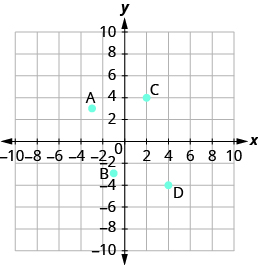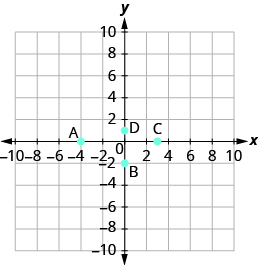## Identifying Points on the Rectangular Coordinate System

### Learning Outcomes

• Name the ordered pair of a point on a coordinate system

In algebra, being able to identify the coordinates of a point shown on a graph is just as important as being able to plot points. To identify the x-coordinate of a point on a graph, read the number on the x-axis directly above or below the point. To identify the y-coordinate of a point, read the number on the y-axis directly to the left or right of the point. Remember, to write the ordered pair using the correct order $\left(x,y\right)$.

### example

Name the ordered pair of each point shown:Solution
Point A is above $-3$ on the $x\text{-axis}$, so the $x\text{-coordinate}$ of the point is $-3$. The point is to the left of $3$ on the $y\text{-axis}$, so the $y\text{-coordinate}$ of the point is $3$. The coordinates of the point are $\left(-3,3\right)$.

Point B is below $-1$ on the $x\text{-axis}$, so the $x\text{-coordinate}$ of the point is $-1$. The point is to the left of $-3$ on the $y\text{-axis}$, so the $y\text{-coordinate}$ of the point is $-3$. The coordinates of the point are $\left(-1,-3\right)$.

Point C is above $2$ on the $x\text{-axis}$, so the $x\text{-coordinate}$ of the point is $2$. The point is to the right of $4$ on the $y\text{-axis}$, so the $y\text{-coordinate}$ of the point is $4$. The coordinates of the point are $\left(2,4\right)$.

Point D is below $4$ on the $x-\text{axis}$, so the $x\text{-coordinate}$ of the point is $4$. The point is to the right of $-4$ on the $y\text{-axis}$, so the $y\text{-coordinate}$ of the point is $-4$. The coordinates of the point are $\left(4,-4\right)$.

### example

Name the ordered pair of each point shown:### try it

Watch the following video for another example of how to determine the ordered pair for points on the coordinate plane.